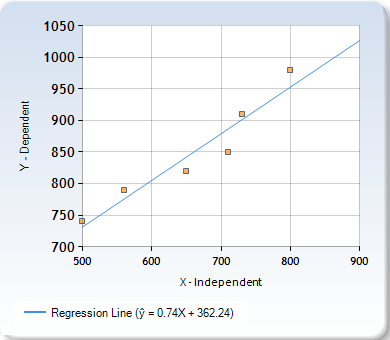## Least Squares Regression Method

### Learning Outcomes

• Use the least-squares regression method to create a regression line on a graph of cost data

This method uses all of the data available to separate the fixed and variable portions of a mixed cost. A regression line is fitted into the data using the following formula:

Y= a + bX

Y= Maintenance costs

X= Number of dogs groomed

a= the total fixed cost

b= the variable cost per unit of activity

So using this calculator.

If you use the data from the dog groomer example you should be able to calculate the following chart:This method is more accurate, using all of the available data. The table below shows the difference in calculation using the two methods:

Costs High-low Least Squares Regression Method
Variable cost estimate per dog groomed $.080$.074
Fixed cost estimate per month $340$362.24

## Contribute!

Did you have an idea for improving this content? We’d love your input.